## Monday, January 31, 2011

### 661

661 is a prime number.

661 has a representation as a sum of two squares: 661 = 62 + 252.

659 and 661 form a twin prime pair.

661 is the hypotenuse of a primitive Pythagorean triple: 6612 = 3002 + 5892.Physicist William Thomson, Lord Kelvin (1824-1907) published 661 papers on a wide range of scientific topics.

## Friday, January 28, 2011

### 4665

4665 = 3 x 5 x 311.

4665 is 33333 in base 6.

4665 is a divisor of 5220 - 1.

The sum of the squares of the digits of 4665 is equal to the largest prime factor reversed, where the largest prime factor is not a palindrome: 42 + 62 + 62 + 52 = 113.IC 4665 is one of the brighter clusters in the Milky Way galaxy.

## Thursday, January 27, 2011

### 460

460 = 22 x 5 x 23.

460 has a unique representation as a sum of three squares: 460 = 62 + 102 + 182.

460 is the sum of twelve consecutive primes: 460 = 17 + 19 + 23 + 29 + 31 + 37 + 41 + 43 + 47 + 53 + 59 + 61.460 Scania is an asteroid discovered in 1900.

Source: Wikipedia

## Wednesday, January 26, 2011

### 3546

3546 = 2 x 32 x 197.

3546 has a representation as a sum of two squares: 3546 = 392 + 452.

3546 is a divisor of 1914 - 1.

3546 is 1414 in base 14.

3546 is a number such that it together with its double and triple contain all digits: 3546, 7092, and 10638.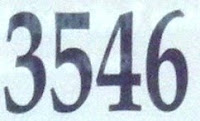3546 is the maximum length of a rook tour on a 17 x 18 board.

## Tuesday, January 25, 2011

### 354

354 = 2 x 3 x 59.

354 is the sum of the first four fourth powers: 354 = 14 + 24 + 34 + 44.

354 is the smallest number whose first two digits are distinct odd primes and whose third digit is the arithmetic mean of the first two.

354 is the sum of two triangular numbers in exactly two ways: 354 = 351 + 3 = 276 + 78.354 is the average number of days in a lunar year (rounded off).

## Monday, January 24, 2011

### 947

947 is a prime number. The difference between any two of its digits is also a prime.

947 is a Chen prime because 947 + 2 = 949 = 13 x 73, so 949 is a semiprime.

947 is the maximum number of regions into which 43 lines divide the plane.

947 is the sum of seven consecutive primes: 947 = 113 + 127 + 131 + 137 + 139 + 149 + 151.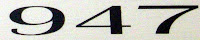947 is the telephone area code for Oakland, Michigan. Note that the words "telephone code Oakland" have, respectively, 9, 4, and 7 digits.

## Friday, January 21, 2011

### 553

553 = 7 x 79.

553 is the sum of nine consecutive primes: 553 = 43 + 47 + 53 + 59 + 61 + 67 + 71 + 73 + 79.

Inserting a zero between adjacent digits of 553 produces a prime: 50503.The CN Tower in Toronto is about 553 meters tall.

## Thursday, January 20, 2011

### 6213

6213 = 3 x 19 x 109.

6213 is a divisor of 466 - 1.

6213 is 44433 in base 6 and 1133 in base 18.

6213 divides the sum of the digits of 216213.

6213 is the first of a triple of integers, each the product of three distinct primes: 6213 = 3 x 19 x 109; 6214 = 2 x 13 x 239; and 6215 = 5 x 11 x 113.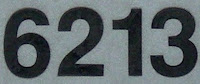The CNR 6213 is a historic Canadian National Railways steam locomotive on display in Toronto.

## Wednesday, January 19, 2011

### 626

626 = 2 x 313. It is the smallest multidigit, palindromic, two-sided semiprime.

626 has a representation as a sum of two squares: 626 = 12 + 252.

626 is a palindrome in base 5 (10001), base 10 (626), and base 16 (272).

626 is the sum of two nonzero fourth powers: 626 = 54 + 14.The Mazda 626 automobile was produced in Japan for the export market.

## Tuesday, January 18, 2011

### 548

548 = 22 x 137.

548 has a representation as a sum of two squares: 548 = 82 + 222.

548 is the maximum number of 9th powers needed to sum to any number.

548 is the sum of a positive square and positive cube in more than one way.

548 is the smallest of three consecutive numbers, each divisible by a square.548 Kressida is an asteroid discovered in 1904.

## Friday, January 14, 2011

### 725

725 = 52 x 29.

725 has three representations as a sum of two squares: 725 = 72 + 262 = 102 + 252 = 142 + 232.

725 is the hypotenuse of two primitive Pythagorean triples: 7252 = 3332 + 6442 = 3642 + 6272.

7252 = 525,625, which involves, 525, 625, and 725.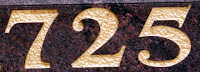The Europcopter EC 725 Super Cougar is a long-range tactical transport helicopter developed for military use.

Source: WolframAlpha

## Thursday, January 13, 2011

### 917

917 = 7 x 131.

917 is the only positive number known whose ninth power can be written as a sum of 10 ninth powers.

917 is the sum of five consecutive primes: 917 = 173 + 179 + 181 + 191 + 193.

917 is a number divisible by the sum of the squares of its digits: 92 + 12 + 72 = 131.The Porsche 917 is a racing car that gave its manufacturer its first overall wins in the 24 Hours of Le Mans race.

## Wednesday, January 12, 2011

### 96

96 = 25 x 3. It is the second smallest number with six prime factors.

96 has a unique representation as a sum of three squares: 96 = 42 + 42 + 82.

96 is 88 in base 11.

96 is the seventh number that stays the same when written upside down.Ninety Six is a town in South Carolina.

Source: Phillips, R. 2004. Numbers: Facts, Figures and Fiction. Badsey Publications.

## Tuesday, January 11, 2011

### 109

109 is a prime number.

109 has a representation as a sum of two squares: 109 = 32 + 102.

107 and 109 form a twin prime pair.

109 is the hypotenuse of a primitive Pythagorean triple: 1092 = 602 + 912.

109 is the smallest number that contains more digits than its square: 1092 = 11881.

109 is the smallest number that is palindromic in bases 5 (414) and 9 (131).109 is the atomic number of the element meitnerium.

## Monday, January 10, 2011

### 8120

8120 = 23 x 5 x 7 x 29.

8120 is a divisor of 414 - 1.

8120 is 4848 in base 12.

8120 is one-third the product of the 10th prime, the previous number, and the following number: 8120 = (28 x 29 x 30)/3.Research in Motion produces the BlackBerry Pearl 8120 smartphone.

## Friday, January 7, 2011

### 801

801 = 32 x 89.

801 has a representation as a sum of two squares: 801 = 152 + 242.

801 = (7! + 8! + 9! + 10!)/(7 x 8 x 9 x 10).

801 is 1441 in base 8 and 171 in base 25.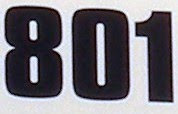801 was an English experimental rock band formed in 1976 for three live concerts.

## Thursday, January 6, 2011

### 245

245 = 5 x 72.

245 has a representation as a sum of two squares: 245 = 72 + 142.

2452 is one of three square numbers using the same digits: 2452 = 60025, 1602 = 25600, and 2502 = 62500.245 is the number of Jewish singers who returned from captivity in Babylon following the rise of Cyrus the Great and the Persian Empire (Nehemiah, chapter 7, verse 67).

## Wednesday, January 5, 2011

### 4884

4884 = 22 x 3 x 11 x 37.

4884 is a divisor of 434 - 1.

66 x 64 = 4224
66 x 67 = 4422
66 x 68 = 4488
66 x 69 = 4554
66 x 74 = 4884

4884 is a palindrome whose sum of prime factors is also a palindrome: 2 + 2 + 3 + 11 + 37 = 55.NGC 4884 is an elliptical galaxy in Coma Berenices.

Source: Numeropedia

## Tuesday, January 4, 2011

### 486

486 = 2 x 35.

486 is a Perrin number.

486 is 200000 in base 3 and 600 in base 9. It is 123 in base 21.

486 is a number with 12 divisors.

486 is the sum of two positive fifth powers: 486 = 35 + 35.Intel's 486 (short for 80486) microprocessor chip powered many microcomputers, starting in 1986.

## Monday, January 3, 2011

### 672

672 = 25 x 3 x 7.

672 has a unique representation as a sum of three squares: 672 = 42 + 162 + 202.

672672 plus the 672nd prime is a prime number.

672 x 276 = 185,472 = 384 x 483.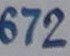672 is the number of hours in February of a non-leap year.